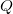# Required Water Pump Horsepower

Use this form to estimate the brake horsepower required. Brake horsepower is the power out of the drive motor, and the power into the water pump and is how most pumps and drive motors are specified. Also calculate the total power requirements (energy use rate). The pump efficiency depends on the pump and the pressure and flow that the pump is operated at and is found on the pump chart from the manufacturer. Drive motor efficiency affects the total power requirements or energy use rate. Motors are usually specified by their power output, not input, so don't use this number to specify the drive motor necessary to drive the pump. For pumps in wells the depth to the free water surface in the well while pumping needs to be added to the pressure required at the surface. Go here to convert this additional depth of water to pressure units. Learn more about the units used on this page.

Pressure:
Flow Rate:
Pump Efficiency:
%
Drive Motor Efficiency:
%
Brake Horsepower:
Total Power Input Requirements (Energy Use Rate):

## The Equations

These formulas calculate the brake horse power and total power. Brake horse power is the power that a brake would have to absorb to stop a motor output and is how most motors (power output) and also pumps (required power in) are specified.Where:= Brake Horse Power (hp)= Flow rate (gpm)= Pressure (psi)= Water Pump Efficiency (decimal)

And:Where:= Drive Motor Efficiency (decimal)

WSU Prosser - IAREC, 24106 N Bunn Rd, Prosser WA 99350-8694, 509-786-2226. Contact Us